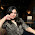## Thursday, 26 July 2012

### CBSE - Class 9 - Science - CH8 - Motion (WorkSheet)

Class 9 - Ch8 - Motion
Fill in the blanks

1. If the position of an object does not change with time, it is said to be at _____.

2. Rest and Motion are ___________ (absolute/relative) terms

3. The study of motion without taking into account the cause of motion is called ______ (kinematics/dynamics).

4. An object is said to be at ________ (rest/motion),  if it changes its position with respect to its surroundings in a given time

5. Distance is the length of ______(actual/shortest) path traveled by a body in a given time.

6. Displacement is the ______ (actual/shortest) path covered by a moving object from the initial point of reference in a specified direction.

7. Distance is a _______ (scalar/vector) physical quantity while displacement is a _______ (scalar/vector) physical quantity.

8. When a body moves unequal distances in equal intervals of time, then the body is said to describe ___________ (uniform/non-uniform) motion.

9. In uniform motion, speed of an object is ________ (constant/not constant).

10. SI unit of velocity is _____ (metre per second/ km per hour/ miles per hour).

11. The rate of change of velocity of a moving body with time is called ______.

12. Slope of position-time graph ________ (is zero/may be zero/ cannot be zero)  if the object is at rest.

13. Slope of the distance-time graph gives the _____ (speed/acceleration) of the object.

14. The nature of distance-time graph is a _______ (straight line/curve) having ______ (uniform/varying) slope when the object has non-uniform motion.

15. The slope of the velocity-time graph gives ______ (displacement/acceleration).

16. An object is under free fall. Considering upward as positive direction, the displacement of the object during a short time interval is positive during ______ (ascent/descent) and negative during _____ (ascent/descent).

17. In a uniform circular motion, velocity of a particle is _____ (constant/not constant) but its speed is ______ (constant/not constant).

Answers:
1. rest.
2. relative
3. kinematics
4. motion
5. actual
6. shortest
7. scalar, vector
8. non-uniform
9. constant
10. metre per second
11. acceleration
12. is zero.
13. speed
14. curve, varying
15. acceleration
16. ascent, descent
17. not constant, constant#### 7 comments:

1.it was nice n helped a lot............................ bt 3rd question was not dere in d ncert book

1.it's not necessary that questions come straight from book

2.true cuz cbse gives u q/a from anywhere

3.2.some questions are there which are not in N.C.E.R.T book

1.that is what the above comment said akshat that there will be answers coming in exams which will not be straight from the book !! questions may also rise for common sense and logic! Example is here in front of u in the 3rd question !! that was also not in the ncert book !!

3.Very nice.

We love to hear your thoughts about this post!